Select Page
MATHESIS :: Essential
• Articles coming soon
• Articles coming soon
• Articles coming soon
• Articles coming soon
• Articles coming soon
• Articles coming soon

# Reasoning by induction

The argument by induction is perhaps the most emblematic of mathematical science and the one that fascinates and disconcerts the most.

It is used when one seeks to prove a property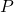(which depends) on the natural numbers, in other words a statement of the form ““.

Such a statement often covers many other relationships between related objects other than the natural numbers, implicit in the formula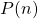.

Reasoning by induction consists in proving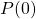, i.e. the propertyfor the value(which is called the induction start), and assuming thatis true (which is called the induction hypothesis), in proving that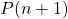is true: this is called the induction step. From “close to close”, this leads to the fact thatis true for any natural number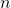.

We reason in this way because the set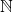being infinite, it is not possible to prove the propertyfor all the natural numbersindividually, i.e.,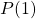,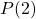, … .

Example 1
The clause: “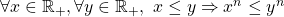“, wheredenotes a generic natural number, is a property ofwhich mentions generic real numbers.

• The propertyis “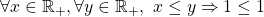“, since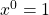for any real number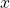• The induction step consists in proving, i.e. “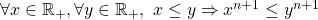“, by assuming.Intuitively, we can compare reasoning by induction to a game of dominoes: we have to make the first domino fall, then each time a domino falls, it drags in its fall the following domino and, step by step, all the dominoes fall.

Similarly, you have to prove the property for the first natural number, and then from one step to the next, each time it is proved for one natural number, it will be true for the next number, if at least you can show the transition from one step to the next, which is often the effort involved in the demonstration.

What makes this possible is that one does not demonstrate each step of the induction individually, but “generically”.

Example 2
Using the same example, let us prove by induction onthat for all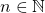,is true. Let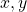be two positive real numbers:

1. Induction start: If, then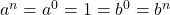by convention, so the inequality holds for2. Induction hypothesis: Suppose thatis true for a natural number, i.e. that3. Induction step: We then have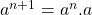(by definition)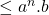(because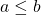and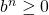)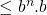(by applying the induction hypothesis and multiplication by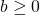)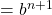4. The property is verified at rank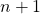, so we have proved by induction that it is verified for all.The justification of this type of argument is based on the induction principle. Indeed, to show that the propertyis true for any natural numberis to prove that the set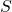of natural numbers for whichis true (symbolically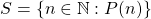), is the set whole set.

For that, one applies the induction principle (REF): one must show that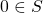(in other words that the propertyis true, initial step), then that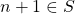as soon as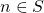(in other words, thatis true as soon asis true, induction step). The principle of demonstration by induction is thus a reformulation of the induction principle!